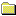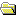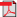Products Download Events Support Videos All Product Families ARM7, ARM9, and Cortex-M3 Products C16x, XC16x, and ST10 Products C251 and 80C251 Products Cx51 and 8051 Products Modified Anytime In the Last 7 Days In the Last 14 Days In the Last 30 Days In the Last 90 Days In the Last 6 Months

Technical Support

On-Line Manuals

Libraries and Floating Point Support GuidePrefaceThe ARM C and C++ LibrariesThe ARM C Micro-libraryFloating-point SupportAbout floating-point supportThe software floating-point library, fplibCalling fplib routinesfplib arithmetic on numbers in a particular formatfplib conversions between floats, long longs, doubfplib comparisons between floats and doublesfplib C99 functionsControlling the ARM floating-point environmentFloating-point functions for compatibility with MiC99-compatible functions for controlling the ARM fC99 rounding mode and floating-point exception macException flag handlingFunctions for handling rounding modesFunctions for saving and restoring the whole floatFunctions for temporarily disabling exceptionsARM floating-point compiler extensions to the C99Writing a custom exception trap handlerExample of a custom exception handlerException trap handling by signalsmathlib double and single-precision floating-pointIEEE 754 arithmeticBasic data types for IEEE 754 arithmeticSingle precision data type for IEEE 754 arithmeticDouble precision data type for IEEE 754 arithmeticSample single precision floating-point values forSample double precision floating-point values forIEEE 754 arithmetic and roundingExceptions arising from IEEE 754 floating-point arException types recognized by the ARM floating-poiUsing the Vector Floating-Point (VFP) support librThe C and C++ Library Functions referenceFloating-point Support Functions Reference

# <!-- var kmNextPage = "armlib_chr1358938942144.htm"; var kmPrevPage = "armlib_chr1358938941598.htm"; var kmNextPageTitle = "fplib conversions between floats, long longs, doub"; var kmPrevPageTitle = "Calling fplib routines"; var kmBreadCrumbs = "<a href=\"default.htm\">Home</a> &raquo; <a href=\"armlib_chr1358938940724.htm\">Floating-point Support</a> &raquo; <a href=\"armlib_chr1358938941317.htm\">The software floating-point library, fplib</a> &raquo; fplib arithmetic on numbers in a particular format"; kmSetupPaging (); kmNavButtons(); //--> fplib arithmetic on numbers in a particular format

### 3.2.2 fplib arithmetic on numbers in a particular format

fplib provides a number of routines to perform arithmetic on numbers in a particular format.

The following table describes these routines. Arguments and return types are always in the same format.

Table 3-1 Arithmetic routines

Function Argument types Return type Operation
`__aeabi_fadd` 2 `float` `float` Return x plus y
`__aeabi_fsub` 2 `float` `float` Return x minus y
`__aeabi_frsub` 2 `float` `float` Return y minus x
`__aeabi_fmul` 2 `float` `float` Return x times y
`__aeabi_fdiv` 2 `float` `float` Return x divided by y
`_frdiv` 2 `float` `float` Return y divided by x
`_frem` 2 `float` `float` Return remainder of x by y (see note a)
`_frnd` `float` `float` Return x rounded to an integer (see note b)
`_fsqrt` `float` `float` Return square root of x
`__aeabi_dadd` 2 `double` `double` Return x plus y
`__aeabi_dsub` 2 `double` `double` Return x minus y
`__aeabi_drsub` 2 `double` `double` Return y minus x
`__aeabi_dmul` 2 `double` `double` Return x times y
`__aeabi_ddiv` 2 `double` `double` Return x divided by y
`_drdiv` 2 `double` `double` Return y divided by x
`_drem` 2 `double` `double` Return remainder of x by y (see notes a and c)ac
`_drnd` `double` `double` Return x rounded to an integer (see note b)b
`_dsqrt` `double` `double` Return square root of x
##### Related information
a
Functions that perform the IEEE 754 remainder operation. This is defined to take two numbers, x and y, and return a number z so that z = x – ny, where n is an integer. To return an exactly correct result, n is chosen so that z is no bigger than half of x (so that z might be negative even if both x and y are positive). The IEEE 754 remainder function is not the same as the operation performed by the C library function `fmod`, where z always has the same sign as x. Where the IEEE 754 specification gives two acceptable choices of n, the even one is chosen. This behavior occurs independently of the current rounding mode.
b
Functions that perform the IEEE 754 round-to-integer operation. This takes a number and rounds it to an integer (in accordance with the current rounding mode), but returns that integer in the floating-point number format rather than as a C `int` variable. To convert a number to an `int` variable, you must use the `_ffix` routines.
c
The IEEE 754 `remainder()` function is a synonym for `_drem`. `remainder()` is defined in math.h.
 Non-ConfidentialPDF version ARM DUI0378H Copyright © 2007, 2008, 2011, 2012, 2014-2016 ARM. All rights reserved.##### Important information

This site uses cookies to store information on your computer. By continuing to use our site, you consent to our cookies.